Select Page

# Regular Expressions In Automata

Regular expressions are used to represent the regular languages in Automata. It is also use in compiler designing.
Regular expressions are just like arithmetic, logic, Boolean expressions.

## Operations on Regular Language

There may various operations on regular languages. Let “R” be a Regular expression over alphabet Sigma if R is

1. If regular expression (R) is equal to Epsilon (ε) then language of Regular expression (R) will represent the epsilon set i.e. { ε}. Mathematical equation is given below,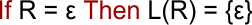2. If regular expression (R) is equal to Φ then language of Regular expression (R) will represent the empty set i.e. { }. Mathematical equation is given below,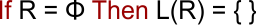3. If regular expression (R) is equal to a input symbol “a” which belongs to sigma, then language of Regular expression (R) will represent the set which having “a” alphabet i.e. {a}. Mathematical equation is given below,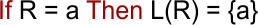4. Union of two Two Regular Expressions will always produce a regular language. Suppose R1 and R2 are two regular expressions. IF R1= a, R2=b then R1 U R2 =a+b So L(R1 U R2) = {a,b}, still string “a,b” is a regular language.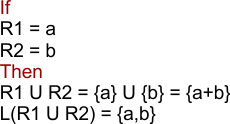Hence, above equation shows that {a,b} is also a regular language.

Note: Intersection of two Two Regular Expressions will always produce a regular language.

5. Concatenation of two Two Regular Expressions will always produce a regular language. IF R1= a, R2=b then R1.R2 =a.b So L(R1.R2) = {ab}, still string “ab” is a regular language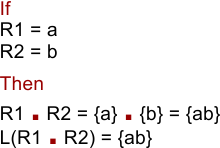Hence, above equation shows that {ab} is also a regular language.

6. Kleene closure of Regular Expression (RE) is also a regular language

If R1 = x and (R1)* is still a regular language

• In a regular expression, x* means zero or more occurrence of x. It can generate { ε, x, xx, xxx, xxxx, …..}
• In a regular expression, x+means one or more occurrence of x. It can generate {x, xx, xxx, xxxx, …..}

7. If R is regular, (R) is also a regular language

Note: Only the above mentioned 7 rules are used for regular expressions. By the combination of above 7-rules more regular Expressions can be created.

## Types of Regular Expressions

As we Know regular languages are of either finite are infinite. So, Regular expressions can be written for both finite and infinite languages. So, types of Regular expressions are of two types

### I. Finite Regular Expressions

Finite Regular expressions are used to represent the finite regular languages. So, the length of finite regular expressions is always limited.

For example,

Write the regular expression for the finite language which accepting all the strings, having the length exactly two over ∑ = {a, b}.

Solution:

Language for given example is given below

L = {aa, ab, ba, bb} // only 4 strings are possible for given condition

Regular expression for above language is given below

L(R) = {aa + ab +ba+bb}

### II. Infinite Regular Expressions

infinite Regular expressions are used to represent the infinite regular languages. So, the length of infinite regular expressions is always unlimited.

For example,

Write the regular expression for the language which accepting all the strings, having the first symbol should be “b” and last symbol should be “a” over ∑ = {a, b}.

Solution:

Language for given example is given below

L = {ba, baa, baba, bbaa, baaaa, babbbba……….. } // unlimited strings are possible for given condition

Regular expression for above language is given below

L(R) = b (a+b)* a

Help Other’s By Sharing…